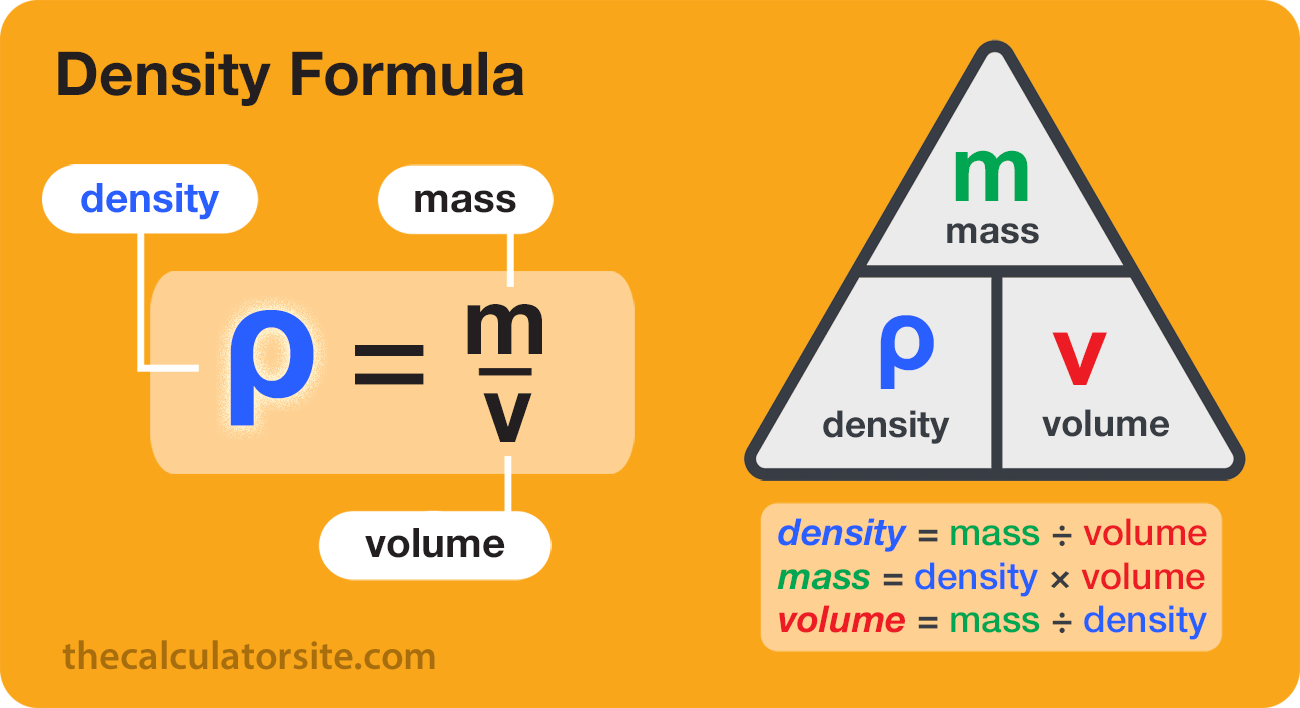# Density Calculator

ρ=m/V
Use this calculator to convert between density, mass and volume. Density is the mass per volume of a substance. Multiple units are available for each calculation option.

Disclaimer: Whilst every effort has been made in building our calculator tools, we are not to be held liable for any damages or monetary losses arising out of or in connection with their use. Full disclaimer.

## What is Density?

Density is a measure of how much mass is contained in a given volume. It describes how tightly packed the particles or molecules of a substance are. Understanding density is important because it helps us explain various phenomena in the physical world, such as why some objects float while others sink, why ice is less dense than liquid water, and why hot air rises.

## Calculating density

The density of a material is scientifically defined as its mass per volume. It can also be thought of as how compacted or compressed a substance is. When calculating a density from a mass and volume figure, it's important to remember that density can be affected by pressure and temperature, particularly where gases are concerned.

Our density calculator uses the density formula: Density (ρ) = Mass (m) ÷ Volume (V).

• Density (ρ) is measured in units such as kilograms per cubic meter (kg/m³), grams per cubic centimeter (g/cm³), or pounds per cubic inch (lb/in³), depending on your preferred unit system.
• Mass (m) is the amount of matter in an object and is typically measured in units such as kilograms (kg) or grams (g).
• Volume (V) is the amount of space an object occupies and is measured in units such as cubic meters (m³) or cubic centimeters (cm³).

To calculate density, divide the mass by the volume. If you enter your two figures for mass and volume into our calculator above, it will return the density.## Calculating mass from density and volume

To calculate mass, simply rearrange the structure of the overall density formula. Multiply your density figure by your volume figure: m = ρ x V

It's a common request that people want to know how they can convert a volume to a weight, hoping it's a simple one-to-one conversion. And it's for that reason that I built my weight to volume converter and cooking calculator tools. So, please do make use of those tools if you need to.

## Calculating volume from mass and density

To calculate volume, rearrange the structure of the density formula in order to divide your mass figure by your density figure : V = m / ρ